### I. Miklós and J. Podani. 2004. Randomization of presence–absence matrices: comments and new algorithms. Ecology 85:86–92.

Appendix B.Sum-of-squares reduction never falls into an infinite cycle

In this appendix, we show that the sum-of-squares reduction never falls into an infinite cycle, namely, if a matrix has row and column totals identical to the row and column totals of a 0-1 matrix, then it can be converted into a 0-1 matrix with quasi-swaps.

Notation: A quasi-swap replacing the submatrix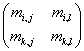by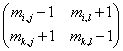is denoted by (ij)(kl).

Theorem Let M = {mi,j} be a nonnegative integer matrix, with row totals a0 = (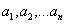) and column totals
b0 = (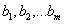).  If a 0-1 matrix exists with the same row and column totals, then M can be transformed into a 0-1 matrix using quasi-swaps.

Proof Let the column totals be ordered decreasing.We prove that if column r has the smallest column total ar, then quasi swaps can transform M into M' in which r has 1’s in the first ar columns. It is enough for the proof, according to the Havel-Hakimi theorem (Havel 1955, Hakimi 1962).

First we prove that entities greater than 1 can be eliminated from row r with quasi-swaps, and then we prove that M' mentioned above can be reached with quasi-swaps, too. Assume that for some column c, mr,c > 1. Let C1 = { c' | mrc’ >0 }, R1 = { r' | mr'c > 0}, R2 = {r'|ar' > 0}\R1, C2 = {c'|bc' > 0}\C1. Note that |C2| > 0 and |R2| > 0 since a 0-1 matrix exists with the same row and column totals. If there are c2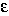C2 and r2R2 with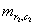>0, then (rc)(r2c2)  is a quasi-swap resulting smaller mr,c. If  "c2C2 and "r2R2= 0, then c1C1 and r2R2 exist such that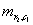>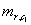since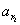³ar. Hence (r2c1)(rc) is an allowed swap resulting in the sum of squared entities decreasing. Since the sum of squared entities cannot be smaller than the sum of the entities, in a finite number of steps we can eliminate all the entities greater than 1 from column c.

Now r has only 0’s and 1’s and we are going to prove the existence of M'. Let u = min{u'| mr,u' = 0, mr,u' + 1 > 0}. There is a r' for which mr',u' > mr',u' + 1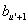£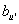. Therefore (ru' + 1)(r'u') is a quasi-swap. By induction on u we can construct the desired M'.

Literature cited

Hakimi, S. 1962. On the realizability of a set of integers as degrees of the vertices of a graph. SIAM Journal of Applied Mathematics 10:496–506.

Havel, V. 1955. A remark on the existence of finite graphs. [In Czech.] Casopis pro Pestováni Matematiky 80:477–480.

[Back to E085-001]